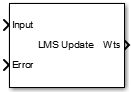Documentation

# LMS Update

Estimate weights of LMS adaptive filter

• Library:
• DSP System Toolbox / Filtering / Adaptive Filters## Description

The LMS Update block estimates the weights of an LMS adaptive filter. The block accepts the data and error as inputs and computes the filter weights based on the algorithm the block chooses. For more details on the algorithms, see Algorithms.

You can use this block to compute the adaptive filter weights in applications such as system identification, inverse modeling, and filtered-x LMS algorithms, which are used in acoustic noise cancellation. For more details, see References.

## Ports

### Input

expand all

Data input to the adaptive filter. The block accepts single-precision or double-precision floating point inputs. All inputs must be scalars and must have the same data type and precision.

Data Types: `single` | `double`
Complex Number Support: Yes

Error between the output signal and the desired signal.

Data Types: `single` | `double`
Complex Number Support: Yes

To enable this port, set Step size source to `Input port`.

Data Types: `single` | `double`

When the input to this port is not zero, the block updates the filter weights. When the input to this port is 0, the filter weights do not change.

Data Types: `single` | `double` | `Boolean` | `int16` | `int32` | `int64` | `int8` | `uint16` | `uint32` | `uint64` | `uint8`

When the input to this port is not zero, the block resets the filter weights to their initial values. When the input to this port is 0, the filter weights do not change.

Data Types: `single` | `double` | `Boolean` | `int16` | `int32` | `int64` | `int8` | `uint16` | `uint32` | `uint64` | `uint8`

### Output

expand all

The length of the filter weights vector is the value in the Filter length parameter.

Data Types: `single` | `double`

## Parameters

expand all

The block uses one of the listed algorithms to compute the filter weights. For more details on the algorithms, see Algorithms.

Filter length specifies the length of the weights vector the block generates through the Wts output port.

• `Property` — Specify the filter adaptation size using the Step size (mu) parameter.

• `Input port` — Pass filter adaptation size using the Mu input port.

Step size (mu) indicates the amount by which the filter weights are updated in each iteration. Choose an optimal step size so that the filter is stable and the convergence speed is optimal.

To enable this parameter, set Step size source to `Property`.

This parameter is tunable. You can change its value even during the simulation.

Leakage factor (0 to 1) prevents unbounded growth of the filter coefficients by reducing the drift of the coefficients from their optimum values. A leakage factor of `1.0` indicates no leakage. If you encounter coefficient drift, that is, large fluctuation about the optimum solution, decrease the leakage factor until the coefficient fluctuation becomes small.

This parameter is tunable. You can change its value even during the simulation.

This parameter specifies the initial value of the filter weights, w(n-1). The block uses this value to compute the weights, w(n), when n = 1. For more details, see Algorithms.

When you select this check box, the Adapt input port appears on the block. When the input to this port is greater than 0, the block updates the filter weights. When the input to this port is less than or equal to 0, the filter weights do not change.

When you select this check box, the Reset input port appears on the block. When the input to this port is greater than 0, the block resets the filter weights to their initial values. When the input to this port is less than or equal to 0, the filter weights do not change.

• `Code generation`

Simulate model using generated C code. The first time you run a simulation, Simulink® generates C code for the block. The C code is reused for subsequent simulations, as long as the model does not change. This option requires additional startup time but provides faster simulation speed than ```Interpreted execution```.

• `Interpreted execution`

Simulate model using the MATLAB®  interpreter. This option shortens startup time but has slower simulation speed than ```Code generation```.

## Block Characteristics

 Data Types `double` | `single` Direct Feedthrough `no` Multidimensional Signals `no` Variable-Size Signals `no` Zero-Crossing Detection `no`

## Algorithms

The block computes filter weight estimates using.

The functionis defined according to the LMS algorithm you specify through the Algorithm parameter:

• `LMS`• `Normalized LMS`In the `Normalized LMS` algorithm, ε is a small positive constant that overcomes the potential numerical instability in the update of weights.

For double-precision floating-point inputs, ε is `2.2204460492503131e-016`. For single-precision floating-point inputs, ε is `1.192092896e-07`. For fixed-point input, ε is 0.

• `Sign-Error LMS`• `Sign-Data LMS`, where u(n) is real

• `Sign-Sign LMS`, where u(n) is real

In the previous equations:

• n — The current time index

• u(n) — The vector of buffered input samples at step n

• u*(n) — The complex conjugate of the vector of buffered input samples at step n

• w(n) — The vector of filter weight estimates at step n

• e(n) — The estimation error at step n

• µ — The adaptation step size

• α — The leakage factor (0 ≤ α ≤ 1)

 Madisetti, Vijay, and Douglas Williams. "Introduction to Adaptive Filters." The Digital Signal Processing Handbook. Boca Raton, FL: CRC Press, 1999.

 Akhtar, M. T., M. Abe, M. Kawamata. "Modified-filtered-x LMS algorithm based active noise control systems with improved online secondary-path modeling." IEEE Symposium on Circuits and Systems, 2004.

Watch now